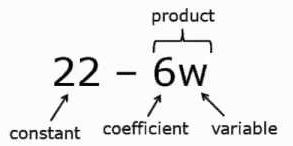# Expression: Term, Factor And Coefficient

An algebraic expression is a mathematical phrase that contains integral or fractional constants (numbers), variables (alphabets) and algebraic operators (such as addition, subtraction, division, multiplication, etc.) operating on them. Also, these expressions are expressed in the form of term, factor and coefficient.

a + 1, a + b, x2 + y, 5x/2, etc. are few examples of the algebraic expressions. The algebraic expressions are readily used as a number of mathematical formulas and find usage in generalizing them.

In this article, you will learn about different elements forming these expressions – terms, its factors and coefficient.## Terms of an Expression

A term can be a number, a variable, product of two or more variables or product of a number and a variable. An algebraic expression is formed by a single term or by a group of terms. For example, in the expression 4x + y, the two terms are 4x and y.

It is to be noted here that terms add up to form an expression. Say there is a term 8xy, which is the product of 8, x and y. There is another term -4z, which is the product of -4 and z. On adding them up, 8xy + (-4z), we get 8xy – 4z, which is an algebraic expression. Based on the variables and their powers in terms, they can be classified into like and unlike algebraic terms of the expression.

## Factor of a Term

• The numbers or variables that are multiplied to form a term are called its factors. Example, 5xy is a term with factors 5, x and y.
• The factors cannot be further factorized. Example, 5xy cannot be written as the product of factors 5 and xy. This is because xy can be factorized to x and y.
• The factors of the term 3a4 are 3, a, a, a and a.
• 1 is not taken as a separate factor.

## Coefficient of a Term

A coefficient is the numerical factor of a term containing constant and variables.

• In the term 5ab, 5 is the coefficient.
• -5 is the coefficient of the term –5ab2.
• When there is no numerical factor in a term, its coefficient is taken as +1. For example, in the term x2y3, the coefficient is +1.
• In the term –x, the coefficient is -1.
• A coefficient is sometimes generalized as either the numerical factor, variable factor or the products of the two. For example, in the term 5ab2, b is the coefficient of 5ab. Similarly, in 10ab, -2a is the coefficient of -5b and so on.

However, all these parts of an algebraic expression are connected with each other by arithmetic operations such as addition, subtraction, or multiplication in general. Thus, these operators play a significant role in forming expressions in algebra. Even the single term can be expressed as a sum of two terms. For example, 5x can also be written as 5x + 0 or 5x – 0. But this way of representation is not actually required except to define the expression as it is a combination of all those elements (i.e. terms, variables, operators, coefficients, and constants).

## What is a Coefficient in Maths?

A coefficient is an integer that is written along with a variable or it is multiplied by the variable. For example, in the term 2x, 2 is the co-efficient.

Those variables which do not carry any number along with them, have a coefficient 1. For example, the term y has coefficient 1.

In linear algebra, when we write the equations, the coefficients always exist for variables.

Practice Questions

Identify the terms, coefficients and variables in each of the following expressions.

1. 22x2 – 6x + 17
2. xy + 2x3 – 14x
3. 3p + 16
4. 15y2 – 19 + 3xy + 4x – y

To learn more about algebraic expressions and related concepts, visit www.byjus.com and also, download BYJU’S – The Learning App to get interactive videos of all the important maths concepts.